# Arithmetic, formal

arithmetical calculus

A logico-mathematical calculus which formalizes elementary number theory. The language of the most common kind of formal arithmetic contains the constant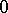, numerical variables, the equality sign, the function symbols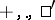(successor) and logical symbols. The terms are built from the constantand the variables of the function symbols; in particular, natural numbers are denoted by terms of the form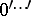. The atomic formulas are equalities of terms; the remaining formulas are built from atomic formulas by means of the logical connectives,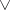,,,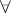,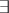. Formulas in the language of formal arithmetic are known as arithmetical formulas. The postulates of formal arithmetic are the postulates of predicate calculus (classical or intuitionistic, depending on the formal arithmetic in question), the Peano axioms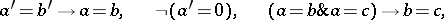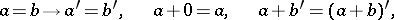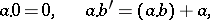and the axiom scheme of induction:where A is an arbitrary formula, known as the induction formula.

The means of formal arithmetic are adequate for the derivation of the theorems presented in standard courses on elementary number theory. At the time of writing (the 1980's) it would appear that no significant number-theoretic theorem which can be demonstrated without recourse to analysis is known which could not be demonstrated by formal arithmetic. To write down and prove such theorems in formal arithmetic, the expressive potential of the latter must be investigated. The expression of many number-theoretic functions in terms of formal arithmetic is especially important. In particular, statements about primitive (and even partial) recursive functions (cf. Recursive function) may be rewritten in the language of formal arithmetic. In this way it is possible to prove formulas expressing important properties of partial recursive functions, in particular their defining equations. Thus, the equation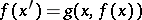is expressed by the formula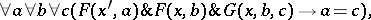whereandare formulas representing the graphs of the functionsand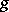, respectively. It is possible to formulate statements about finite sets in the language of formal arithmetic. Moreover, classical formal arithmetic is equivalent to the Zermelo–Fraenkel axiomatic set theory without the axiom of infinity: A model of any one of these systems can also be constructed in the other system.

The deductive force of the system of formal arithmetic is characterized by the ordinal(the least solution of the equation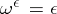): It is possible to deduce in formal arithmetic the scheme of transfinite induction up to any ordinal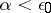, but not the scheme of induction up to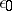. The class of provably-recursive functions of the system of formal arithmetic (i.e. the class of partial recursive functions, the general recursiveness of which can be established by the means of formal arithmetic) coincides with the class of ordinal recursive functions with ordinals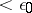. This makes it possible to extend formal arithmetic in certain ways, e.g. to have a formal arithmetic with symbols for all primitive recursive functions and the corresponding additional postulates. Formal arithmetic satisfies both Gödel incompleteness theorems (cf. Gödel incompleteness theorem). In particular, there exist polynomials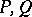in several variables such that the formula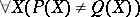cannot be proved even though expressing a true statement — the consistency of the system of formal arithmetic. A combinatorial (Ramsey-type) theorem independent of formal arithmetic has been constructed .

In studying the structure of a system (in particular, the problems of consistency), formal arithmetic is formulated without quantifiers, but with Hilbert's-symbol. The formulas of this system are quantifier-free, but it is permitted to use terms of the type(yielding somewhich satisfies conditionif it exists; otherwise). The postulates of the-system are the postulates of propositional calculus, the equality rules, the rule of substitution for the free numerical variables, the axioms for the functions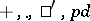(predecessor), and the following-axioms: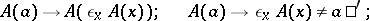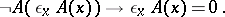The quantifiers are introduced by means of abbreviations:The induction axiom turns out to be derivable.

The formulas of formal arithmetic with free variables define number-theoretic predicates. Formulas not containing free variables (closed formulas) express propositions. A-place predicateabout natural numbers is called arithmetic if there is an arithmetical formulasuch that for any natural numbers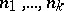, the relation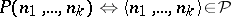is valid. This determines a classification of arithmetic predicates by the type of prefix in the prenex form of the respective formula. The class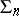(the class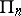) consists of predicatesrepresentable by a formula of the formwhereis a primitive recursive function,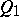is(or, respectively,) andare alternating quantifiers (i.e.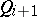iswhere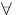is, and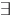is). Each such class contains a universal predicate, i.e. a predicatesuch that for each one-place predicate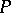of this class there exists a number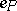for which the identity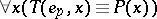is valid. Multi-place predicates can be reduced to one-place predicates with the aid of functions which code systems of natural numbers. For anythe inequalityis valid, and a class with a lower index is properly included in any class with a larger index. This classification of predicates generates a classification of arithmetic functions based on the classification of their graphs. Not all number-theoretic predicates are arithmetic: an example of a non-arithmetic predicate is the predicate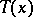such that for any closed arithmetical formulathe identityis valid, where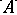is the number of the formulain some fixed numbering satisfying some natural conditions. The predicateplays an important role in the studies of the structure of formal arithmetic, in particular in the problem of the independence of its axioms. The addition to formal arithmetic of the symbolwith axioms of the type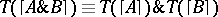, which express its commutativity with logical connectives, makes it possible to prove that formal arithmetic is consistent. The same construction, this time inside formal arithmetic, may be carried out for a subsystemof formal arithmetic in which the induction scheme is restricted by the following condition: The complexity of the induction formula is, i.e. it belongs to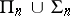. The function ofis successfully performed by, say, the universal predicate for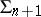, and the corresponding proof is performed in the system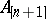. It follows from Gödel's second incompleteness theorem thatis stronger than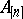, so that formal arithmetic does not coincide with any one of the systems, and the induction scheme cannot be replaced by any finite set of axioms. Formal arithmetic turns out to be complete with respect to formulas from the class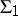: A closed formula of this class is provable in formal arithmetic if and only if it is true. Since the classcontains an algorithmically-unsolvable predicate, it follows that the problem of provability in formal arithmetic is algorithmically unsolvable.

If formal arithmetic is defined as the Gentzen formal system of the usual type, a cut (the analogue of modus ponens in Gentzen systems) cannot be eliminated. Elimination of a cut becomes possible if the induction scheme is replaced by the rule of infinite induction (-rule):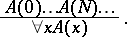One of the first proofs of the consistency of formal arithmetic was obtained in this way. A manageable formulation of the-rule is: "The number e is a number of the general recursive function which describes the derivation of length <0 by the w-rule" (-proof). This predicate belongs to the class, and the system obtained is not formal, but only semi-formal. To each derivation of a formulain formal arithmetic it is possible to assign a numbersuch that the statement "e is an w-proof of the formula A without a cut" is true, and can even be proved in formal arithmetic. Since a cut-free-proof of a closed quantifier-free formula contains no quantifiers, it follows that formal arithmetic is consistent. If Gödel's second incompleteness theorem is now applied, it follows that the cut elimination theorem from any proof in formal arithmetic cannot be demonstrated by the means of formal arithmetic; nevertheless, for any given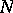a proof in formal arithmetic is possible for any derivation with complexity of induction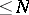. Passing to-proofs makes it possible to establish many meta-mathematic theorems about the system of formal arithmetic, in particular completeness with respect to formulas ofand the ordinal character of provably-recursive functions.